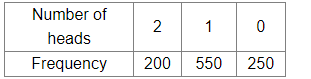# Two coins are tossed 1000 times and the outcomes are recorded as given below:

Question:

Two coins are tossed 1000 times and the outcomes are recorded as given below:Now, if two coins are tossed at random, what is the probability of getting at most one head?

(a) $\frac{3}{4}$

(b) $\frac{4}{5}$

(c) $\frac{1}{4}$

(d) $\frac{1}{5}$

Solution:

Total number of times two coins are tossed = 1000

Number of times of getting at most one head = Number of times of getting 0 heads + Number of times of getting 1 head = 250 + 550 = 800

$\therefore P($ Getting at most one head $)=\frac{\text { Number of times of getting at most one head }}{\text { Total number of times two coins are tossed }}=\frac{800}{1000}=\frac{4}{5}$

Hence, the correct answer is option (b).